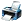# Please solve the following problems using dimensional analysis

747 views

(i) Convert 14 mm to its equivalent in m.
(ii) Convert 35 kg to its equivalent in g.
(iii) Convert 57 mL to its equivalent in L.
(iv) Convert a speed of 88 m/s to its equivalent in cm/s.
(v) Convert a density of 9.45 g/L to its equivalent in g/mL.
(vi) The density of mercury metal is 1.6 g/mL. What is the mass of 3.55 mL of the metal?
(vii) The density of lead is 11.3 g/mL. What is the mass of 45 mL of the metal?
(viii) The density of table salt, NaCl, is 2.16 g/mL. what is the mass of 100.0 mL of this solid?
(ix) A particle moves through a gas at a speed of 15 km/s. How far will it move in 5.5 s?
(x) A mole of copper contains 6.02×1023 atoms. How many atoms are there in 0.525 moles?
(xi) A solution of barium nitrate contains 61.2 g per liter of solution. How many grams of barium nitrate is
contained in 2.75 L of this solution?
(xii) A sample of seawater contains 0.000245 g of sodium chloride per mL of solution. How much sodium
chloride is contained in 50.0 mL of this solution?posted Jul 15, 2017
All these problems are same . These are problems on unit conversion, not of dimentional analytic problems at all . Please read the "Dimentions and Units" from any text on physics of H.S. standart, preferably NCERT texts .

One example is given for you : 14 mm=24×(1mm/1m)×1m=14×(1mm/1000mm)×1m=0.014×1m=0.014m .
All others are same . But you must know the conversion factors .answer Aug 21, 2017 by

Hello,
Dimensional analysis is a very important part of physics according to the JEE point of view
The Unit and dimension covers around 3.50 % weightage in JEE exams
You can take help from the following books to prepare for JEE physics
NCERT
H.C VERMA for physics
DC PANDEY for class physics
Check out the JEE Advanced Syllabus Nowanswer Sep 12, 2017
Similar Questions

Hello,

IIT JEE is one of the toughest examination in World because of its low admission rates which is around 9,369 in 4,79,651 in year 2012. So if you are thinking to Crack JEE Mains then you must go for JEE Mains tricks 2018 - https://scoop.eduncle.com/jee-mains-preparation-tips

Getting right guidance for the preparation of JEE Mains Exam is very important. Time management & strategies play a crucial role in any exam preparation. It becomes more important when you are preparing for JEE Mains without coaching.